# Class 8 NCERT Solutions – Chapter 11 Mensuration – Exercise 11.1

• Last Updated : 24 Nov, 2020

### Question 1. A square and a rectangular field with measurements as given in the figure have the same perimeter. Which field has a larger area?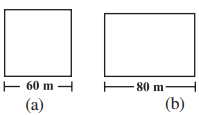Solution:

Attention reader! All those who say programming isn't for kids, just haven't met the right mentors yet. Join the  Demo Class for First Step to Coding Coursespecifically designed for students of class 8 to 12.

The students will get to learn more about the world of programming in these free classes which will definitely help them in making a wise career choice in the future.

Given,

Side of a square = 60 m

Length, l = 80 m

According to question,

Perimeter of rectangular field = Perimeter of square field

According to formula,

2(l + b) = 4 × Side

2(80 + b) = 4 × 60

160 + 2b = 240

b = 40

Therefore, Breadth of the rectangle is 40 m.

Now, Area of Square field = (side)2 = (60)2 = 3600 m2

And Area of Rectangular field = length × breadth = 80 × 40 = 3200 m2

Hence, area of square field is larger.

### Question 2. Mrs.Kaushik has a square plot with the measurement as shown in the figure. She wants to construct a house in the middle of the plot. A garden is developed around the house. Find the total cost of developing a garden around the house at the rate of Rs. 55 per m2.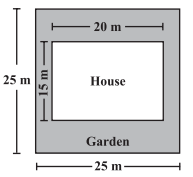Solution:

Given,

Side of a square = 25 m

According to formula,

Area of square plot =  square of a side = (side)2 = (25)2 = 625

Therefore, the area of a square plot is 625 m2

Length of the house = 20 m and

Breadth of the house = 15 m

Area of the house = length × breadth = 20 × 15 = 300 m2

Area of garden = Area of square plot – Area of house = 625 – 300 = 325 m2

Therefore, per unit cost of making garden is Rs. 55

Total Cost of developing the garden of 325 sq. m = Rs. 55 × 325 = Rs. 17,875

### Question 3. The shape of a garden is rectangular in the middle and semi-circular at the ends as shown in the diagram. Find the area and the perimeter of this garden [Length of rectangle is 20 – (3.5 + 3.5 meters)]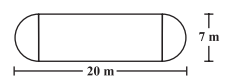Solution:

Given,

Total length = 20 m

Diameter of semi circle = 7 m

Therefore, Radius of semi circle = 7/2 = 3.5 m

Length of rectangular field = 20 – (3.5 + 3.5) = 20 – 7 = 13 m

Breadth of the rectangular field = 7 m

Area of rectangular field = l × b = 13 × 7= 91m2

Area of two semi circles = 2 × (1/2) × pi × r2

= 2 × (1/2) × 22/7 × 3.5 × 3.5

= 38.5 m2

Area of garden = 91 + 38.5 = 129.5 m2

Now Perimeter of two semi circles =  2.pi.r = 2 × (22/7) × 3.5  = 22 m

Therefore, Perimeter of garden = 22 + 13 + 13 = 48 m

### Question 4. A flooring tile has the shape of a parallelogram whose base is 24 cm and the corresponding height is 10 cm. How many such tiles are required to cover a floor of area 1080 m2? [If required you can split the tiles in whatever way you want to fill up the corners]

Solution:

Given,

Base of flooring tile = 24 cm = 0.24 m

Corresponding height of a flooring tile = 10 cm = 0.10 m

Now Area of flooring tile = Base × Altitude = 0.24 × 0.10 = 0.024

Area of flooring tile is 0.024m2

Number of tiles required to cover the floor = Area of floor/Area of one tile = 1080/0.024 = 45000 tiles

Hence 45000 tiles are required to cover the floor.

### Question 5. An ant is moving around a few food pieces of different shapes scattered on the floor. For which food-piece would the ant have to take a longer round? Remember, the circumference of a circle can be obtained by using the expression C = 2.pi.r,wherer is the radius of the circle.

Solution:

(a)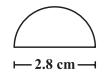Radius =  Diameter/2 = 2.8/2 cm = 1.4 cm

Circumference of semi-circle = pi.r = (22/7)×1.4 = 4.4

Circumference of semi-circle is 4.4 cm

Total distance covered by the ant = Circumference of semi – circle + Diameter = 4.4 + 2.8 = 7.2 cm

(b)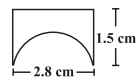Diameter of semi-circle = 2.8 cm

Radius =  Diameter/2 = 2.8/2  = 1.4 cm

Circumference of semi-circle = r = (22/7) × 1.4 = 4.4 cm

Total distance covered by the ant= 1.5 + 2.8 + 1.5 + 4.4 = 10.2 cm

(c)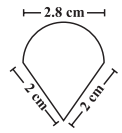Diameter of semi-circle = 2.8 cm

Radius =  Diameter/2 = 2.8/2 = 1.4 cm

Circumference of semi-circle =  pi.r =  (22/7) × 1.4 = 4.4 cm

Total distance covered by the ant = 2 + 2 + 4.4 = 8.4 cm

After analyzing results of three figures, we concluded that for figure (b) food piece, the ant would take a longer round.

My Personal Notes arrow_drop_up